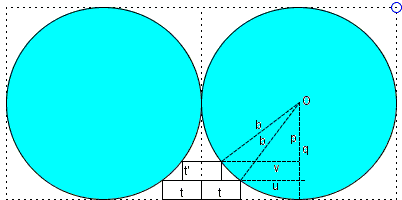# Three Squares and Two Ellipses

Two congruent ellipses with the major axis 2a and the minor axis 2b touch each other, the minor axis of each passing through the point of contact; l is a common exterior tangent parallel to the minor axis.There are three congruent squares of side t such that two of the squares have a side in common and another side lying on l, and each has a vertex on one of the ellipses, and a third square has a vertex on each ellipse and a side that lies along a side of the first two squares.

Show that

a = 2b and t = a/5.

The problem was carved on an 1838 tablet in the Aichi prefecture. The tablet has since disappeared, but the solution has been discussed in 1976 by H. Fukagawa with a reference to a 1837(!) publication, Chosyu Sinpeki by Tameyuki Yosida. The title translates as Tablets in the Chosyu Prefecture.

Solution

### References

1. H. Fukagawa, D. Pedoe, Japanese Temple Geometry Problems, The Charles Babbage Research Center, Winnipeg, 1989 (pp. 62, 154-155)

Write to:

Charles Babbage Research Center
P.O. Box 272, St. Norbert Postal Station
Winnipeg, MB### Solution

The calculations that are necessarily a part of any solution are greatly simplified by an affine trasnformation that squeezes the diagram towards l with coefficient b/a. The horizontal dimensions remain the same, whereas all vertical dimensions are mulitplied by b/a. In particular, the ellipses convert to the circles of radius b and the t×t squares become rectangles t'×t, with t' = tb/a.In the diagram,

q = b - t', p = b - 2t', u = b - t, v = b - t/2.

From

b² = q² + u² = (b - bt/a)² + (b - t)²

it follows that

 (1) 2bt²/a = b² - 2bt(1 + b/a) + t²(1 + b/a)²

so that (very clever, I must say)

 (1') t √2b/a  = b - (1 + b/a)t.

Again, from

b² = v² + p² = (b - t/2)² + (b - 2bt/a)²

we can deduce that

 (2) 2bt²/a = b² - 2bt(1/2 + 2b/a) + t²(1/2 + 2b/a)²

so that

 (2') t √2b/a  = b - (1/2 + 2b/a)t.

From (1') and (2'),

1 + b/a = 1/2 + 2b/a,

that is, a = 2b. From (1'), t = a/2 - 3t/2, or 5t = a.

Note: the steps from (1) to (1') and from (2) to (2') are based on unproven assumptions that the right hand sides in (1) and (2) are positive. If they are not, the derivations amount to claiming that 5² = (-5)², say, implies 5 = -5. Which is of course absurd.## Sangaku### Conic Sections > Ellipse